# DreamBooth

Author: Sayak Paul, Chansung Park
Date created: 2023/02/01
Description: Implementing DreamBooth.View in ColabGitHub source

## Introduction

In this example, we implement DreamBooth, a fine-tuning technique to teach new visual concepts to text-conditioned Diffusion models with just 3 - 5 images. DreamBooth was proposed in DreamBooth: Fine Tuning Text-to-Image Diffusion Models for Subject-Driven Generation by Ruiz et al.

DreamBooth, in a sense, is similar to the traditional way of fine-tuning a text-conditioned Diffusion model except for a few gotchas. This example assumes that you have basic familiarity with Diffusion models and how to fine-tune them. Here are some reference examples that might help you to get familiarized quickly:

``````!pip install -q -U keras_cv
!pip install -q -U tensorflow
``````

If you're running the code, please ensure you're using a GPU with at least 24 GBs of VRAM.

## Initial imports

``````import math

import keras_cv
import matplotlib.pyplot as plt
import numpy as np
import tensorflow as tf
from imutils import paths
from tensorflow import keras
``````

## Usage of DreamBooth

... is very versatile. By teaching Stable Diffusion about your favorite visual concepts, you can

• Recontextualize objects in interesting ways:• Generate artistic renderings of the underlying visual concept:And many other applications. We welcome you to check out the original DreamBooth paper in this regard.

DreamBooth uses a technique called "prior preservation" to meaningfully guide the training procedure such that the fine-tuned models can still preserve some of the prior semantics of the visual concept you're introducing. To know more about the idea of "prior preservation" refer to this document.

Here, we need to introduce a few key terms specific to DreamBooth:

• Unique class: Examples include "dog", "person", etc. In this example, we use "dog".
• Unique identifier: A unique identifier that is prepended to the unique class while forming the "instance prompts". In this example, we use "sks" as this unique identifier.
• Instance prompt: Denotes a prompt that best describes the "instance images". An example prompt could be - "f"a photo of {unique_id} {unique_class}". So, for our example, this becomes - "a photo of sks dog".
• Class prompt: Denotes a prompt without the unique identifier. This prompt is used for generating "class images" for prior preservation. For our example, this prompt is - "a photo of dog".
• Instance images: Denote the images that represent the visual concept you're trying to teach aka the "instance prompt". This number is typically just 3 - 5. We typically gather these images ourselves.
• Class images: Denote the images generated using the "class prompt" for using prior preservation in DreamBooth training. We leverage the pre-trained model before fine-tuning it to generate these class images. Typically, 200 - 300 class images are enough.

In code, this generation process looks quite simply:

``````from tqdm import tqdm
import numpy as np
import hashlib
import keras_cv
import PIL
import os

class_images_dir = "class-images"
os.makedirs(class_images_dir, exist_ok=True)

model = keras_cv.models.StableDiffusion(img_width=512, img_height=512, jit_compile=True)

class_prompt = "a photo of dog"
num_imgs_to_generate = 200
for i in tqdm(range(num_imgs_to_generate)):
images = model.text_to_image(
class_prompt,
batch_size=3,
)
idx = np.random.choice(len(images))
selected_image = PIL.Image.fromarray(images[idx])
hash_image = hashlib.sha1(selected_image.tobytes()).hexdigest()
image_filename = os.path.join(class_images_dir, f"{hash_image}.jpg")
selected_image.save(image_filename)
``````

To keep the runtime of this example short, the authors of this example have gone ahead and generated some class images using this notebook.

Note that prior preservation is an optional technique used in DreamBooth, but it almost always helps in improving the quality of the generated images.

``````instance_images_root = tf.keras.utils.get_file(
origin="https://huggingface.co/datasets/sayakpaul/sample-datasets/resolve/main/instance-images.tar.gz",
untar=True,
)
class_images_root = tf.keras.utils.get_file(
origin="https://huggingface.co/datasets/sayakpaul/sample-datasets/resolve/main/class-images.tar.gz",
untar=True,
)
``````

## Visualize images

First, let's load the image paths.

``````instance_image_paths = list(paths.list_images(instance_images_root))
class_image_paths = list(paths.list_images(class_images_root))
``````

Then we load the images from the paths.

``````def load_images(image_paths):
images = [np.array(keras.utils.load_img(path)) for path in image_paths]
return images
``````

And then we make use a utility function to plot the loaded images.

``````def plot_images(images, title=None):
plt.figure(figsize=(20, 20))
for i in range(len(images)):
ax = plt.subplot(1, len(images), i + 1)
if title is not None:
plt.title(title)
plt.imshow(images[i])
plt.axis("off")
``````

Instance images:

``````plot_images(load_images(instance_image_paths[:5]))
``````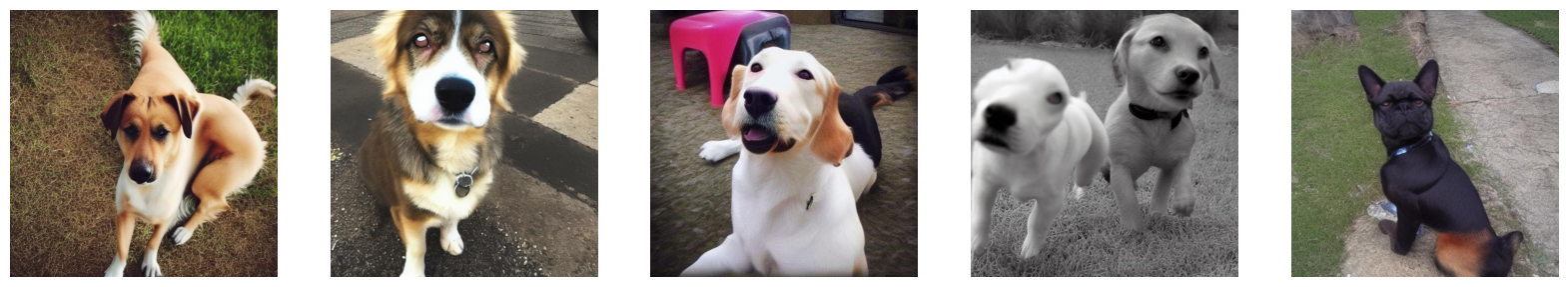Class images:

``````plot_images(load_images(class_image_paths[:5]))
``````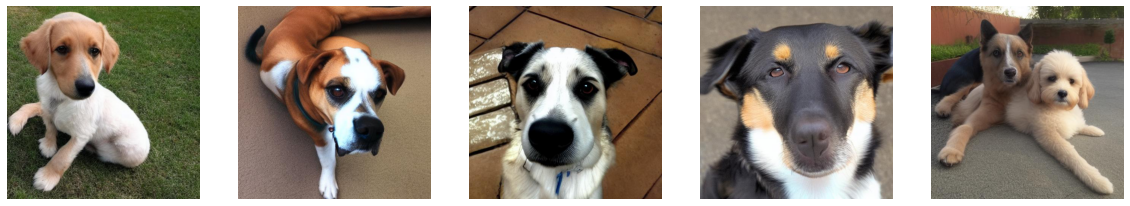## Prepare datasets

Dataset preparation includes two stages: (1): preparing the captions, (2) processing the images.

### Prepare the captions

``````# Since we're using prior preservation, we need to match the number
# of instance images we're using. We just repeat the instance image paths
# to do so.
new_instance_image_paths = []
for index in range(len(class_image_paths)):
instance_image = instance_image_paths[index % len(instance_image_paths)]
new_instance_image_paths.append(instance_image)

# We just repeat the prompts / captions per images.
unique_id = "sks"
class_label = "dog"

instance_prompt = f"a photo of {unique_id} {class_label}"
instance_prompts = [instance_prompt] * len(new_instance_image_paths)

class_prompt = f"a photo of {class_label}"
class_prompts = [class_prompt] * len(class_image_paths)
``````

Next, we embed the prompts to save some compute.

``````import itertools

# The padding token and maximum prompt length are specific to the text encoder.
# If you're using a different text encoder be sure to change them accordingly.
max_prompt_length = 77

tokenizer = keras_cv.models.stable_diffusion.SimpleTokenizer()

# Method to tokenize and pad the tokens.
def process_text(caption):
tokens = tokenizer.encode(caption)
tokens = tokens + [padding_token] * (max_prompt_length - len(tokens))
return np.array(tokens)

# Collate the tokenized captions into an array.
tokenized_texts = np.empty(
(len(instance_prompts) + len(class_prompts), max_prompt_length)
)

for i, caption in enumerate(itertools.chain(instance_prompts, class_prompts)):
tokenized_texts[i] = process_text(caption)

# We also pre-compute the text embeddings to save some memory during training.
POS_IDS = tf.convert_to_tensor([list(range(max_prompt_length))], dtype=tf.int32)
text_encoder = keras_cv.models.stable_diffusion.TextEncoder(max_prompt_length)

gpus = tf.config.list_logical_devices("GPU")

# Ensure the computation takes place on a GPU.
# Note that it's done automatically when there's a GPU present.
# This example just attempts at showing how you can do it
# more explicitly.
with tf.device(gpus.name):
embedded_text = text_encoder(
[tf.convert_to_tensor(tokenized_texts), POS_IDS], training=False
).numpy()

# To ensure text_encoder doesn't occupy any GPU space.
del text_encoder
``````

## Prepare the images

``````resolution = 512
auto = tf.data.AUTOTUNE

augmenter = keras.Sequential(
layers=[
keras_cv.layers.CenterCrop(resolution, resolution),
keras_cv.layers.RandomFlip(),
keras.layers.Rescaling(scale=1.0 / 127.5, offset=-1),
]
)

def process_image(image_path, tokenized_text):
image = tf.io.decode_png(image, 3)
image = tf.image.resize(image, (resolution, resolution))
return image, tokenized_text

def apply_augmentation(image_batch, embedded_tokens):
return augmenter(image_batch), embedded_tokens

def prepare_dict(instance_only=True):
def fn(image_batch, embedded_tokens):
if instance_only:
batch_dict = {
"instance_images": image_batch,
"instance_embedded_texts": embedded_tokens,
}
return batch_dict
else:
batch_dict = {
"class_images": image_batch,
"class_embedded_texts": embedded_tokens,
}
return batch_dict

return fn

def assemble_dataset(image_paths, embedded_texts, instance_only=True, batch_size=1):
dataset = tf.data.Dataset.from_tensor_slices((image_paths, embedded_texts))
dataset = dataset.map(process_image, num_parallel_calls=auto)
dataset = dataset.shuffle(5, reshuffle_each_iteration=True)
dataset = dataset.batch(batch_size)
dataset = dataset.map(apply_augmentation, num_parallel_calls=auto)

prepare_dict_fn = prepare_dict(instance_only=instance_only)
dataset = dataset.map(prepare_dict_fn, num_parallel_calls=auto)
return dataset
``````

## Assemble dataset

``````instance_dataset = assemble_dataset(
new_instance_image_paths,
embedded_text[: len(new_instance_image_paths)],
)
class_dataset = assemble_dataset(
class_image_paths,
embedded_text[len(new_instance_image_paths) :],
instance_only=False,
)
train_dataset = tf.data.Dataset.zip((instance_dataset, class_dataset))
``````

## Check shapes

Now that the dataset has been prepared, let's quickly check what's inside it.

``````sample_batch = next(iter(train_dataset))
print(sample_batch.keys(), sample_batch.keys())

for k in sample_batch:
print(k, sample_batch[k].shape)

for k in sample_batch:
print(k, sample_batch[k].shape)
``````
``````dict_keys(['instance_images', 'instance_embedded_texts']) dict_keys(['class_images', 'class_embedded_texts'])
instance_images (1, 512, 512, 3)
instance_embedded_texts (1, 77, 768)
class_images (1, 512, 512, 3)
class_embedded_texts (1, 77, 768)
``````

During training, we make use of these keys to gather the images and text embeddings and concat them accordingly.

## DreamBooth training loop

Our DreamBooth training loop is very much inspired by this script provided by the Diffusers team at Hugging Face. However, there is an important difference to note. We only fine-tune the UNet (the model responsible for predicting noise) and don't fine-tune the text encoder in this example. If you're looking for an implementation that also performs the additional fine-tuning of the text encoder, refer to this repository.

``````import tensorflow.experimental.numpy as tnp

class DreamBoothTrainer(tf.keras.Model):
# Reference:
# https://github.com/huggingface/diffusers/blob/main/examples/dreambooth/train_dreambooth.py

def __init__(
self,
diffusion_model,
vae,
noise_scheduler,
use_mixed_precision=False,
prior_loss_weight=1.0,
**kwargs,
):
super().__init__(**kwargs)

self.diffusion_model = diffusion_model
self.vae = vae
self.noise_scheduler = noise_scheduler
self.prior_loss_weight = prior_loss_weight

self.use_mixed_precision = use_mixed_precision
self.vae.trainable = False

def train_step(self, inputs):
instance_batch = inputs
class_batch = inputs

instance_images = instance_batch["instance_images"]
instance_embedded_text = instance_batch["instance_embedded_texts"]
class_images = class_batch["class_images"]
class_embedded_text = class_batch["class_embedded_texts"]

images = tf.concat([instance_images, class_images], 0)
embedded_texts = tf.concat([instance_embedded_text, class_embedded_text], 0)
batch_size = tf.shape(images)

# Project image into the latent space and sample from it.
latents = self.sample_from_encoder_outputs(self.vae(images, training=False))
# Know more about the magic number here:
# https://keras.io/examples/generative/fine_tune_via_textual_inversion/
latents = latents * 0.18215

# Sample noise that we'll add to the latents.
noise = tf.random.normal(tf.shape(latents))

# Sample a random timestep for each image.
timesteps = tnp.random.randint(
0, self.noise_scheduler.train_timesteps, (batch_size,)
)

# Add noise to the latents according to the noise magnitude at each timestep
# (this is the forward diffusion process).
tf.cast(latents, noise.dtype), noise, timesteps
)

# Get the target for loss depending on the prediction type
# just the sampled noise for now.
target = noise  # noise_schedule.predict_epsilon == True

# Predict the noise residual and compute loss.
timestep_embedding = tf.map_fn(
lambda t: self.get_timestep_embedding(t), timesteps, dtype=tf.float32
)
model_pred = self.diffusion_model(
[noisy_latents, timestep_embedding, embedded_texts], training=True
)
loss = self.compute_loss(target, model_pred)
if self.use_mixed_precision:
loss = self.optimizer.get_scaled_loss(loss)

# Update parameters of the diffusion model.
trainable_vars = self.diffusion_model.trainable_variables
if self.use_mixed_precision:

return {m.name: m.result() for m in self.metrics}

def get_timestep_embedding(self, timestep, dim=320, max_period=10000):
half = dim // 2
log_max_preiod = tf.math.log(tf.cast(max_period, tf.float32))
freqs = tf.math.exp(
-log_max_preiod * tf.range(0, half, dtype=tf.float32) / half
)
args = tf.convert_to_tensor([timestep], dtype=tf.float32) * freqs
embedding = tf.concat([tf.math.cos(args), tf.math.sin(args)], 0)
return embedding

def sample_from_encoder_outputs(self, outputs):
mean, logvar = tf.split(outputs, 2, axis=-1)
logvar = tf.clip_by_value(logvar, -30.0, 20.0)
std = tf.exp(0.5 * logvar)
sample = tf.random.normal(tf.shape(mean), dtype=mean.dtype)
return mean + std * sample

def compute_loss(self, target, model_pred):
# Chunk the noise and model_pred into two parts and compute the loss
# on each part separately.
# Since the first half of the inputs has instance samples and the second half
# has class samples, we do the chunking accordingly.
model_pred, model_pred_prior = tf.split(
model_pred, num_or_size_splits=2, axis=0
)
target, target_prior = tf.split(target, num_or_size_splits=2, axis=0)

# Compute instance loss.
loss = self.compiled_loss(target, model_pred)

# Compute prior loss.
prior_loss = self.compiled_loss(target_prior, model_pred_prior)

# Add the prior loss to the instance loss.
loss = loss + self.prior_loss_weight * prior_loss
return loss

def save_weights(self, filepath, overwrite=True, save_format=None, options=None):
# Overriding this method will allow us to use the `ModelCheckpoint`
# callback directly with this trainer class. In this case, it will
# only checkpoint the `diffusion_model` since that's what we're training
# during fine-tuning.
self.diffusion_model.save_weights(
filepath=filepath,
overwrite=overwrite,
save_format=save_format,
options=options,
)

def load_weights(self, filepath, by_name=False, skip_mismatch=False, options=None):
# Similarly override `load_weights()` so that we can directly call it on
# the trainer class object.
filepath=filepath,
by_name=by_name,
skip_mismatch=skip_mismatch,
options=options,
)
``````

## Trainer initialization

``````# Comment it if you are not using a GPU having tensor cores.
tf.keras.mixed_precision.set_global_policy("mixed_float16")

use_mp = True  # Set it to False if you're not using a GPU with tensor cores.

image_encoder = keras_cv.models.stable_diffusion.ImageEncoder(resolution, resolution)
dreambooth_trainer = DreamBoothTrainer(
diffusion_model=keras_cv.models.stable_diffusion.DiffusionModel(
resolution, resolution, max_prompt_length
),
# Remove the top layer from the encoder, which cuts off the variance and only
# returns the mean.
vae=tf.keras.Model(
image_encoder.input,
image_encoder.layers[-2].output,
),
noise_scheduler=keras_cv.models.stable_diffusion.NoiseScheduler(),
use_mixed_precision=use_mp,
)

# These hyperparameters come from this tutorial by Hugging Face:
# https://github.com/huggingface/diffusers/tree/main/examples/dreambooth
learning_rate = 5e-6
beta_1, beta_2 = 0.9, 0.999
weight_decay = (1e-2,)
epsilon = 1e-08

learning_rate=learning_rate,
weight_decay=weight_decay,
beta_1=beta_1,
beta_2=beta_2,
epsilon=epsilon,
)
dreambooth_trainer.compile(optimizer=optimizer, loss="mse")
``````

## Train!

We first calculate the number of epochs, we need to train for.

``````num_update_steps_per_epoch = train_dataset.cardinality()
max_train_steps = 800
epochs = math.ceil(max_train_steps / num_update_steps_per_epoch)
print(f"Training for {epochs} epochs.")
``````
``````Training for 4 epochs.
``````

And then we start training!

``````ckpt_path = "dreambooth-unet.h5"
ckpt_callback = tf.keras.callbacks.ModelCheckpoint(
ckpt_path,
save_weights_only=True,
monitor="loss",
mode="min",
)
dreambooth_trainer.fit(train_dataset, epochs=epochs, callbacks=[ckpt_callback])
``````
``````Epoch 1/4
200/200 [==============================] - 301s 462ms/step - loss: 0.1203
Epoch 2/4
200/200 [==============================] - 94s 469ms/step - loss: 0.1139
Epoch 3/4
200/200 [==============================] - 94s 469ms/step - loss: 0.1016
Epoch 4/4
200/200 [==============================] - 94s 469ms/step - loss: 0.1231

<keras.callbacks.History at 0x7f19726600a0>
``````

## Experiments and inference

We ran various experiments with a slightly modified version of this example. Our experiments are based on this repository and are inspired by this blog post from Hugging Face.

First, let's see how we can use the fine-tuned checkpoint for running inference.

``````# Initialize a new Stable Diffusion model.
dreambooth_model = keras_cv.models.StableDiffusion(
img_width=resolution, img_height=resolution, jit_compile=True
)

# Note how the unique identifier and the class have been used in the prompt.
prompt = f"A photo of {unique_id} {class_label} in a bucket"
num_imgs_to_gen = 3

images_dreamboothed = dreambooth_model.text_to_image(prompt, batch_size=num_imgs_to_gen)
plot_images(images_dreamboothed, prompt)
``````
``````By using this model checkpoint, you acknowledge that its usage is subject to the terms of the CreativeML Open RAIL-M license at https://raw.githubusercontent.com/CompVis/stable-diffusion/main/LICENSE
50/50 [==============================] - 42s 160ms/step
``````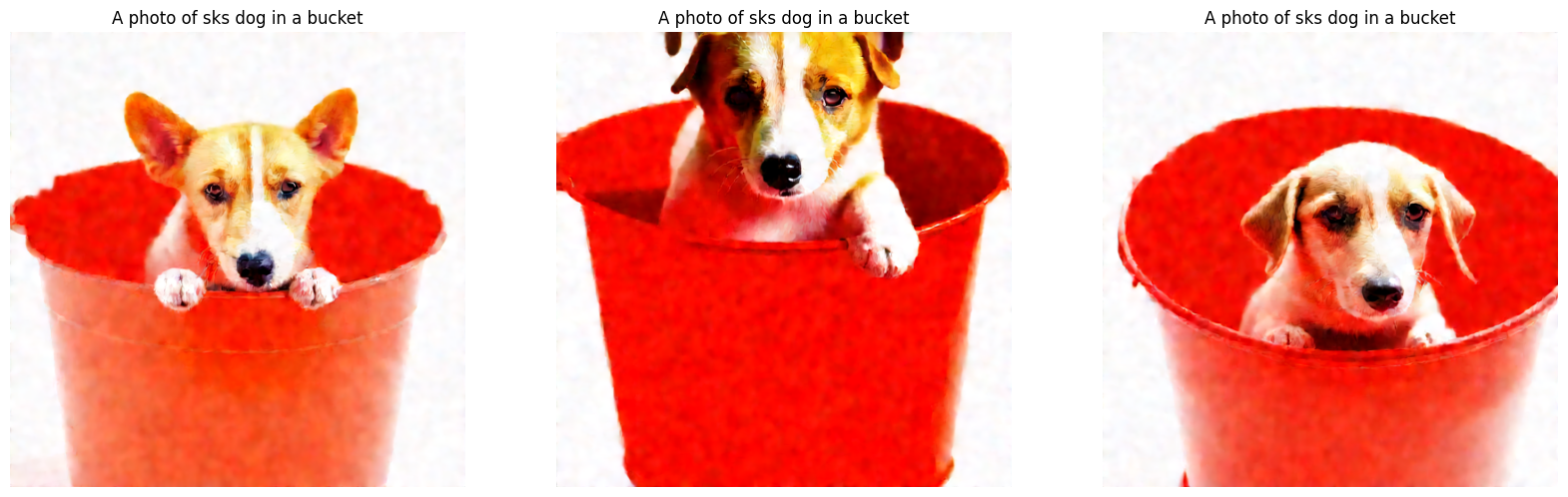Now, let's load checkpoints from a different experiment we conducted where we also fine-tuned the text encoder along with the UNet:

``````unet_weights = tf.keras.utils.get_file(
origin="https://huggingface.co/chansung/dreambooth-dog/resolve/main/lr%409e-06-max_train_steps%40200-train_text_encoder%40True-unet.h5"
)
text_encoder_weights = tf.keras.utils.get_file(
origin="https://huggingface.co/chansung/dreambooth-dog/resolve/main/lr%409e-06-max_train_steps%40200-train_text_encoder%40True-text_encoder.h5"
)

images_dreamboothed = dreambooth_model.text_to_image(prompt, batch_size=num_imgs_to_gen)
plot_images(images_dreamboothed, prompt)
``````
``````Downloading data from https://huggingface.co/chansung/dreambooth-dog/resolve/main/lr%409e-06-max_train_steps%40200-train_text_encoder%40True-unet.h5
3439088208/3439088208 [==============================] - 67s 0us/step
492466760/492466760 [==============================] - 9s 0us/step
50/50 [==============================] - 8s 159ms/step
``````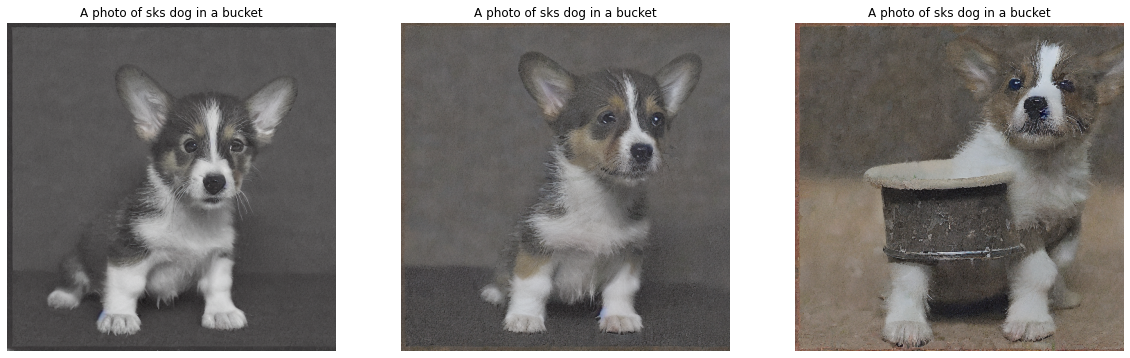The default number of steps for generating an image in `text_to_image()` is 50. Let's increase it to 100.

``````images_dreamboothed = dreambooth_model.text_to_image(
prompt, batch_size=num_imgs_to_gen, num_steps=100
)
plot_images(images_dreamboothed, prompt)
``````
``````100/100 [==============================] - 16s 159ms/step
``````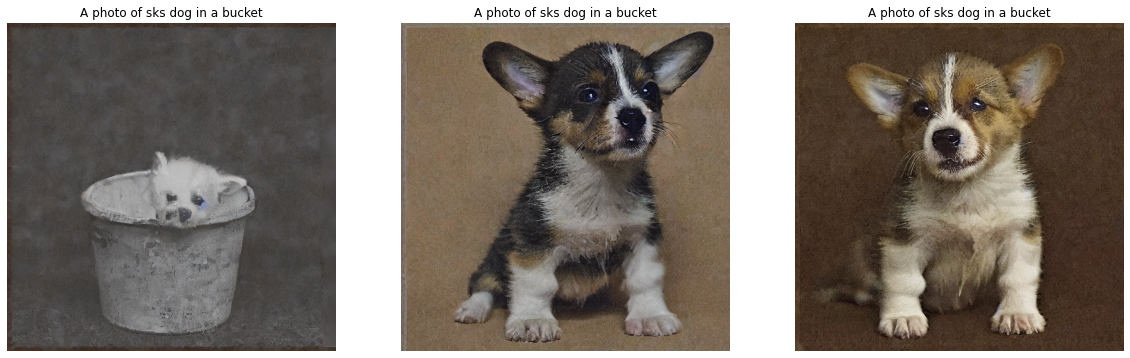Feel free to experiment with different prompts (don't forget to add the unique identifer and the class label!) to see how the results change. We welcome you to check out our codebase and more experimental results here. You can also read this blog post to get more ideas.

## Acknowledgements

• Thanks to the DreamBooth example script provided by Hugging Face which helped us a lot in getting the initial implementation ready quickly.
• Getting DreamBooth to work on human faces can be challenging. We have compiled some general recommendations here. Thanks to Abhishek Thakur for helping with these.